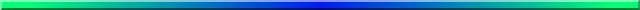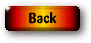# Randomization TestsWe will begin with randomization tests, because they are closer in intent to more traditional parametric tests than are bootstrapping procedures. Their primary goal is to test some null hypothesis, although that null is distinctly different from what it would be with a parametric test. I have probably beaten this dead horse too much, but I will take one more whack at it.

In parametric tests we randomly sample from one or more populations. We make certain assumptions about those populations, most commonly that they are normally distributed with equal variances. We establish a null hypothesis that is framed in terms of parameters, often of the form m1 -m2 = 0 . We use our sample statistics as estimates of the corresponding population parameters, and calculate a test statistic (such as a t test). We then refer that test statistic to the tabled sampling distribution of the statistic, and reject the null if our test statistic is extreme relative to the tabled distribution.

Randomization tests differ from parametric tests in almost every respect.

• There is no requirement that we have random samples from one or more populations—in fact we usually have not sampled randomly.
• We rarely think in terms of the populations from which the data came, and there is no need to assume anything about normality or homoscedasticity.
• Our null hypothesis has nothing to do with parameters, but is phrased rather vaguely, as, for example, the hypothesis that the treatment has no effect on the how participants perform.
• That might be phrased a bit more precisely by saying that, under the null hypothesis, the score that is associated with a participant is independent of the treatment that person received.
• Because we are not concerned with populations, we are not concerned with estimating (or even testing) characteristics of those populations.
• We do calculate some sort of test statistic, however we do not compare that statistic to tabled distributions.
• Instead, we compare it to the results we obtain when we repeatedly randomize the data across the groups, and calculate the corresponding statistic for each randomization.
• Even more than parametric tests, randomization tests emphasize the importance of random assignment of participants to treatments.

To elaborate on the previous points, suppose that we had two groups of participants who were randomly assigned to treatments. One treatment was a control condition with scores of 17, 21, and 23 on some dependent variable, and the other was an intervention condition with scores of 22, 25, 25, and 26. It is not important where the participants came from, so long as they were randomized across treatments. If the treatment had no effect on scores, the first number that we sampled (17) could just as easily have been found for the second treatment as for the first. With 3 observations in the Control condition, and 4 observations in the intervention condition, and if the null hypothesis is true, any 3 of those 7 observations could equally well have landed in control condition, with the remainder landing in the intervention condition. The data are "exchangeable" between conditions. After calculating all of the possible combinations of the 7 observations into one group of 3 and another group of 4 (there are 35 such arrangements), we calculate the relevant test statistic for each arrangement, and compare our obtained statistic to that reference distribution (usually referred to as a sampling distribution with parametric tests). We then reject or retain the null. In this case, you will find that there is only one arrangement of the data that would have a smaller mean for treatment 1 and a larger mean for treatment 2. Thus, for a one tailed test, there are only two data sets (including the one we obtained) that are at least as extreme as the data we found. So a difference as great as ours would occur only 2 times out of 35, for a probability of .0571 under the null hypothesis. (The corresponding two-tailed test would have a probability of approximately .1142.)

Cliff Lunneborg has written an excellent discussion of randomization tests. (Unfortunately, Cliff Lunneborg has died, and the paper is no longer available at his web site. By some good fortune, I happen to have copies of those pages. I have finally made them available for direct downloading at LunneborgPapers.zip. I consider this required reading to understand the underlying issues behind randomization tests. Lunneborg writes extremely well, but (and?) he chooses his words very carefully. Don't read this when you are too tired to do anything else--you have to be alert.

At this point it might be useful to skim the first part of the page on a randomization test between the means of two samples. If you do so, you will see that the example includes data from two conditions in which we record the amount of time that it took someone to leave their parking place once they arrived at their car. In one condition another driver was waiting for the space, and in the other condition there was no one waiting. The question concerns whether the presence of someone who wants the parking space affects the time that it takes the parked driver to leave. Although what follows refers to randomization tests in general, I need to focus on an example, and the example that I have chosen involves differences between two independent groups.

### The Null Hypothesis

As I have said, the issue of the null hypothesis is a bit murkier with nonparametric tests in general than it is with parametric tests. At the very least we replace specific terms like "mean" with loose generic terms like "location". And we generally substitute some vague statement, such as "having someone waiting will not affect the time it takes to back out," for precise statements like "m1 = m2." This vagueness gains us some flexibility, but it also makes the interpretation of the test more difficult. I elaborated on this issue in the section on the philosophy of resampling procedures.

### Basic Approach

The basic approach to randomization tests is straightforward. I'll use the two independent group example, but any other example would do about as well.

• Decide on a metric to measure the effect in question.
• For this example I will use the t statistic, though several others are possible and equivalent, including the difference between the means or the mean of the first group. (Most discussions of this specific test would focus on the difference between means, but I will stick with the traditional Student's t test because that makes for a better parallel between randomization and parametric tests.)
• Calculate that test statistic on the data (here denoted tobt).
• Repeat the following N times, where N is a number greater than 1000
• Shuffle the data

• Assign the first n1 observations to the first condition, and the remaining n2 observations to the second condition.

• Calculate the test statistic (here denoted ti*) for the reshuffled data.

• If ti* is greater than tobt increment a counter by 1.

• I would normally use absolute values, because I want a two-tailed test.

• Continue this procedure N times.

• Divide the value in the counter by N, to get the proportion of times the t on the randomized data exceeded the tobt on the data we actually obtained.
• This is the probability of such an extreme result under the null.
• Reject or retain the null on the basis of this probability.

This approach can be taken with any randomization test. We simply need to modify it to shuffle the appropriate values and calculate the appropriate test statistic. For example, with multiple conditions we will shuffle the data, assign the first n1 cases to treatment 1, the next n2 cases to treatment 2, and so on, calculate an F statistic on the data, consider whether or not to increment the counter, reshuffle the data, calculate F, and so on. In some cases, the hardest question to answer is "What should be shuffled?"

### Terminology

A name for tests such as the one I just described, which has been around for some time, is "permutation test." It refers to the fact that with randomization tests we permute the data into all sorts of different orders, and then calculate our test statistic on each permutation. (One problem with this name, as I see it, is that we aren't really taking permutations--we are taking different combinations. Take an example of two groups with scores 3, 6, 7 and 5, 8, 9. We want to examine all possible ways of assigning 3 of those six values of group one, and the rest to group two. But we don't distinguish the case where group one had 3, 8, 9 from the case where it had 8, 9, 3. These are the same combination, and will give the same mean, median, variance, etc. So it is the different combinations, not permutations, that we care about. I could call them "combination tests," but I would be the only one who did, and I'd look pretty funny hanging out there all by myself.)

The phrase "permutation (or combination) test" has another implication that we need to worry about. It implies, without stating it, that we take all possible permutations. That is often practically impossible, as we will see in a minute.

The phrase "randomization test" is a nice compromise, because it avoids the awkwardness of "permutation," and doesn't suggest anything about the number of samples. It is also very descriptive. We randomize (i.e., randomly order) our data, and then calculate our statistic on those randomized data. I will try to restrict myself to that label.

### The Monte Carlo approach

In the previous section I suggested that the phrase "permutation test" implies that we take all possible permutations of the data (or at least all possible combinations). That is often quite impossible. Suppose that we have three groups with 20 observations per group. There are 60!/(20!*20!*20!) possible different combinations of those observations into three groups, and that means 5.7783*1026 combinations, and even the fastest supercomputer is not up to drawing all of those samples. I suppose that it could be done if we really had to do it, but it certainly wouldn't be worth waiting around all that time for the answer.

The solution is that we take a random sample of all possible combinations. That random sample won't produce an exact answer, but it will be so close that it won't make any difference. The results of 5000 samples will certainly be close enough to the exact answer to satisfy any reasonable person. (The difference will come in the 3rd decimal place or beyond). (There is even a sense in which that approach can be claimed to be exact, but we won't go there.)

When we draw random samples to estimate the result of drawing all possible samples, we often refer to that process as Monte Carlo sampling. I could use that term to distinguish between cases where the number of combinations is small enough to draw all of them, and cases where that is not practical. However there is little to be gained by adding another term, and I will simply use the phrase "randomization tests" for both approaches.

You are probably getting tired of a general discussion that does not focus on a specific example. It is time to return to the main resampling page that move on to such an example. We will start by asking about differential effects for two groups.

### References

Efron, B. & Tibshirani, R. J. (1993) An introduction to the bootstrap. New York: Chapman and Hall.

Lunneborg, C. E. (2000) Random assignment of available cases: Let the inference fit the design. http://faculty.washington.edu/lunnebor/Australia/randomiz.p dfLast revised: 03/01/2007